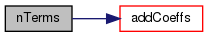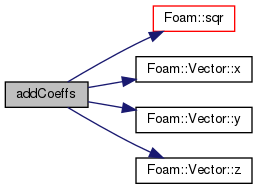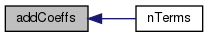The OpenFOAM Foundation

Quadratic polynomial for centred interpolation fitting. More...

## Static Public Member Functions

static label nTerms (const direction dim)

static void addCoeffs (scalar *coeffs, const vector &d, const scalar weight, const direction dim)

## Detailed Description

Quadratic polynomial for centred interpolation fitting.

Can be used with the CentredFit scheme to create a quadratic surface interpolation scheme

Definition at line 48 of file quadraticFitPolynomial.H.

## ◆ nTerms()

 static label nTerms ( const direction dim )
inlinestatic

Definition at line 54 of file quadraticFitPolynomial.H.

Here is the call graph for this function:static void addCoeffs ( scalar * coeffs, const vector & d, const scalar weight, const direction dim )
inlinestatic

Definition at line 65 of file quadraticFitPolynomial.H.

References Foam::sqr(), Vector< Cmpt >::x(), Vector< Cmpt >::y(), and Vector< Cmpt >::z().